login  home  contents  what's new  discussion  bug reports help  links  subscribe  changes  refresh  edit

fricas
(1) -> Z==>Integer; Q==>Fraction Z
Type: Void
fricas
z: Symbol := 'z; P==>UnivariatePolynomial(z,Q)
Type: Void
fricas
t:P := monomial(1,1)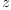(1)
Type: UnivariatePolynomial(z,Fraction(Integer))
fricas
p:P := (1-t)*(1-t^2)*(1-t^3)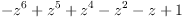(2)
Type: UnivariatePolynomial(z,Fraction(Integer))
fricas
L==>UnivariateLaurentSeries(Q,z,0)
Type: Void
fricas
R ==> Record(k: Z, c: Q)
Type: Void
fricas
l: List R := reverse [[degree m, leadingCoefficient m]$R for m in monomials p]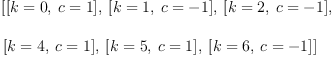(3) Type: List(Record(k: Integer,c: Fraction(Integer))) fricas series(l)$L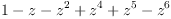(4)
Type: UnivariateLaurentSeries?(Fraction(Integer),z,0)

fricas
l1: List R := [[n, if n=5 then 0 else n/1]$R for n in 1..7]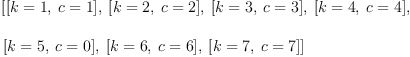(5) Type: List(Record(k: Integer,c: Fraction(Integer))) fricas series(l1)$L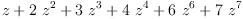(6)
Type: UnivariateLaurentSeries?(Fraction(Integer),z,0)

It seems weird that the resulting series is aborted at a non-existing coefficient in the input list.

fricas
l2: List R := [[n, n/1]$R for n in 1..7|n~=5]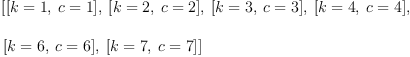(7) Type: List(Record(k: Integer,c: Fraction(Integer))) fricas series(l2)$L(8)
Type: UnivariateLaurentSeries?(Fraction(Integer),z,0)

There is obviously also a bug here, because the zero coefficient should have been removed. I would, however, accept such a result if the specification of series were made precise as to rely on the input stream not to contain zero coefficients.

fricas
S ==> SparseUnivariateLaurentSeries(Q,z,0)
Type: Void
fricas
series(l)$S(9) Type: SparseUnivariateLaurentSeries?(Fraction(Integer),z,0) fricas series(l1)$S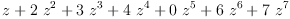(10)
Type: SparseUnivariateLaurentSeries?(Fraction(Integer),z,0)
fricas
series(l2)\$S(11)
Type: SparseUnivariateLaurentSeries?(Fraction(Integer),z,0)

 Subject:   Be Bold !! ( 14 subscribers )